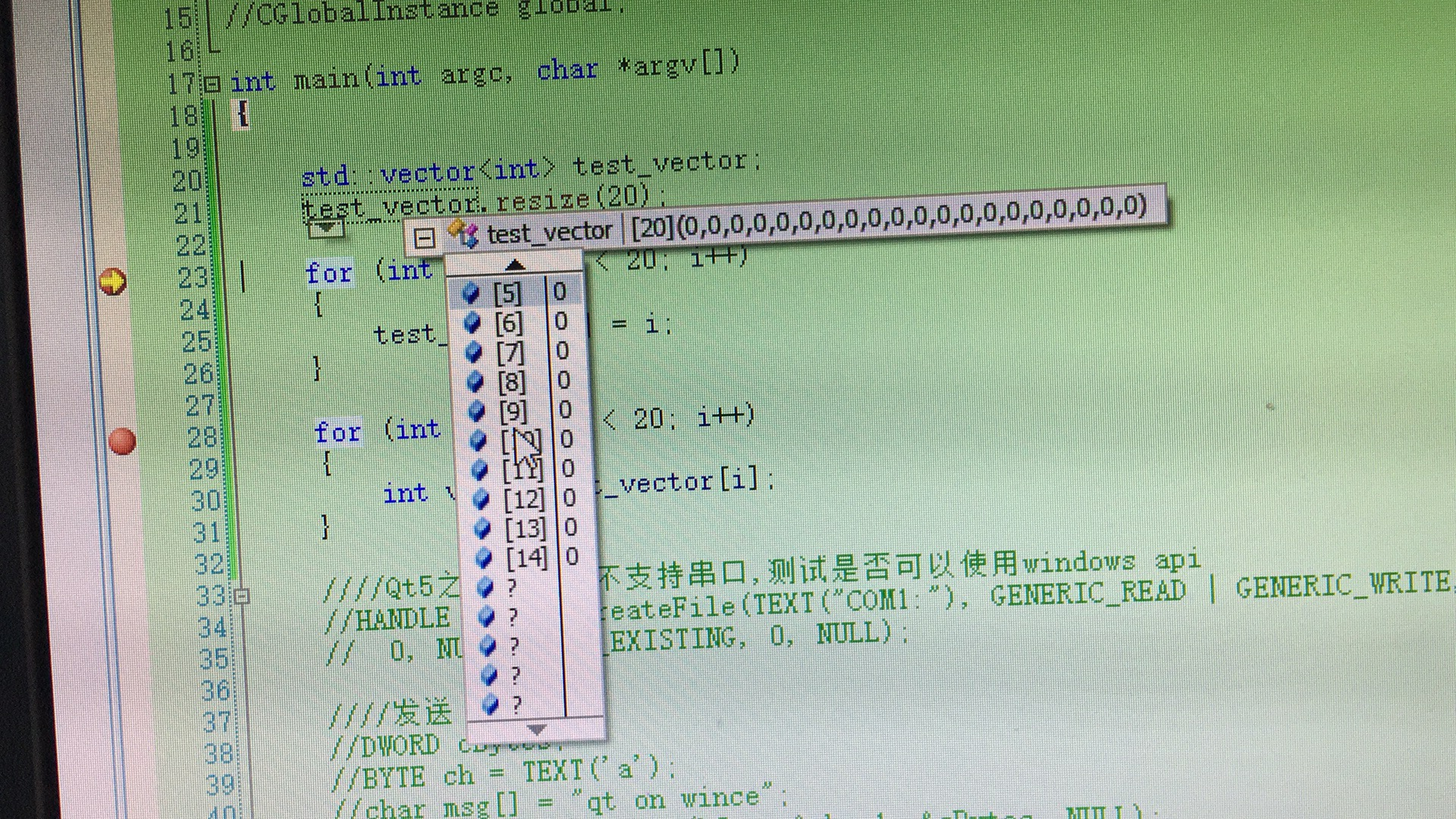# wince vector problem, i need help

• ``````std::vector<int> test1;
test1.resize(15);
int sz = test1.size();
for (int i = 0; i < sz; i++)
{
test1[i] = i;
}

for (int i = 0; i < sz; i++)
{
int val = test1[i];
}

std::vector<int> test2;
test2.resize(20);
sz = test2.size();
for (int i = 0; i < sz; i++)
{
test1[i] = i;
}

for (int i = 0; i < sz; i++)
{
int val = test1[i];
}
``````

error: index out of range
IDE: vs2008
QT: qt4.7.3 for everywhere
https://forum.qt.io/topic/19049/qt-under-windows-ce-is-very-slow

•• std::vector<int> test2;
test2.resize(20);
sz = test2.size();
for (int i = 0; i < sz; i++)
{
test1[i] = i;
}

for (int i = 0; i < sz; i++)
{
int val = test1[i];
}

sz = test2.size() ==> and in loop you are using test1.

`test1` has only 15 elements!

`````` for (int i = 0; i < test1.size(); i++)
{
test1[i] = i;
}
``````

•https://forum.qt.io/topic/19049/qt-under-windows-ce-is-very-slow

still error, vector index out of range

i used qt4.8 wince, still this error,

• std::vector<int> test2;
test2.resize(20);
sz = test2.size();
for (int i = 0; i < sz; i++)
{
test2[i] = i;
}

for (int i = 0; i < sz; i++)
{
int val = test2[i];
}

sorry , i write wrong, but still error

• @CraigTao just to clarify `std::vector` has nothing to do with Qt, it is from the C++ compiler standard library: std::vector

• @CraigTao In which line exactly does that happen? And did you try to debug step by step to see what happens and what values all your variables have?

• Neither has it anything particular to do with mobile and embedded systems

• @KroMignon I Use QVector instead of std::vector , this error still here,

• @CraigTao Then please use debugger to debug and see what happens.
You did not even tell us in which line exactly this happens...